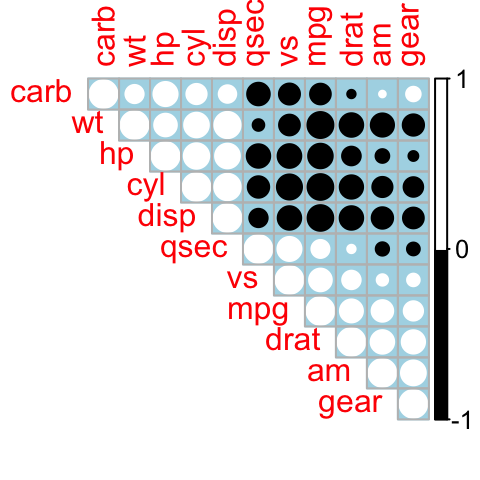# Introduction

This article describes how to plot a correlogram in R. Correlogram is a graph of correlation matrix. It is very useful to highlight the most correlated variables in a data table. In this plot, correlation coefficients is colored according to the value. Correlation matrix can be also reordered according to the degree of association between variables. The R corrplot package is used here.

Note that online software is also available here to compute correlation matrix and to plot a correlogram without any installation.

# Install R corrplot package

corrplot package is required to execute the R code in this article.

``install.packages("corrplot")``

# Data for correlation analysis

The mtcars data is used to compute correlation matrix.

``head(mtcars)``
``````                   mpg cyl disp  hp drat    wt  qsec vs am gear carb
Mazda RX4         21.0   6  160 110 3.90 2.620 16.46  0  1    4    4
Mazda RX4 Wag     21.0   6  160 110 3.90 2.875 17.02  0  1    4    4
Datsun 710        22.8   4  108  93 3.85 2.320 18.61  1  1    4    1
Hornet 4 Drive    21.4   6  258 110 3.08 3.215 19.44  1  0    3    1
Hornet Sportabout 18.7   8  360 175 3.15 3.440 17.02  0  0    3    2
Valiant           18.1   6  225 105 2.76 3.460 20.22  1  0    3    1``````

# Computing correlation matrix

``````M<-cor(mtcars)
``````       mpg   cyl  disp    hp  drat    wt  qsec    vs    am  gear  carb
mpg   1.00 -0.85 -0.85 -0.78  0.68 -0.87  0.42  0.66  0.60  0.48 -0.55
cyl  -0.85  1.00  0.90  0.83 -0.70  0.78 -0.59 -0.81 -0.52 -0.49  0.53
disp -0.85  0.90  1.00  0.79 -0.71  0.89 -0.43 -0.71 -0.59 -0.56  0.39
hp   -0.78  0.83  0.79  1.00 -0.45  0.66 -0.71 -0.72 -0.24 -0.13  0.75
drat  0.68 -0.70 -0.71 -0.45  1.00 -0.71  0.09  0.44  0.71  0.70 -0.09
wt   -0.87  0.78  0.89  0.66 -0.71  1.00 -0.17 -0.55 -0.69 -0.58  0.43``````

# Correlogram : Visualizing the correlation matrix

R corrplot function is used to plot the graph of the correlation matrix.

The simplified format of the function is :

``corrplot(corr, method="circle")``
Arguments Description
corr The correlation matrix to visualize. To visualize a general matrix, please use is.corr=FALSE.
method The visualization method : “circle”, “color”, “number”, etc.

## Visualization methods

Seven different visualization methods can be used : “circle”, “square”, “ellipse”, “number”, “shade”, “color”, “pie”.

``````library(corrplot)
corrplot(M, method="circle")````````corrplot(M, method="pie")````corrplot(M, method="color")``Positive correlations are displayed in blue and negative correlations in red color. Color intensity and the size of the circle are proportional to the correlation coefficients.

Display the correlation coefficient :

``corrplot(M, method="number")``## Types of correlogram layout

There are three types of layout :

• “full” (default) : display full correlation matrix
• “upper”: display upper triangular of the correlation matrix
• “lower”: display lower triangular of the correlation matrix
``corrplot(M, type="upper")````corrplot(M, type="lower")``## Reordering the correlation matrix

The correlation matrix can be reordered according to the correlation coefficient. This is important to identify the hidden structure and pattern in the matrix. “hclust” for hierarchical clustering order is used in the following examples.

``````# correlogram with hclust reordering
corrplot(M, type="upper", order="hclust")````````````# Using different color spectrum
col<- colorRampPalette(c("red", "white", "blue"))(20)
corrplot(M, type="upper", order="hclust", col=col)````````````# Change background color to lightblue
corrplot(M, type="upper", order="hclust", col=c("black", "white"),
bg="lightblue")``````## Changing the color of the correlogram

As shown in the above section, the color of the correlogram can be customized. RcolorBrewer palette of colors are used in the R script below :

``````library(RColorBrewer)
corrplot(M, type="upper", order="hclust",
col=brewer.pal(n=8, name="RdBu"))````````````corrplot(M, type="upper", order="hclust",
col=brewer.pal(n=8, name="RdYlBu"))````````````corrplot(M, type="upper", order="hclust",
col=brewer.pal(n=8, name="PuOr"))``````## Changing the color and the rotation of text labels

tl.col (for text label color) and tl.srt (for text label string rotation) are used to change text colors and rotations.

``corrplot(M, type="upper", order="hclust", tl.col="black", tl.srt=45)``## Combining correlogram with the significance test

### Computing the p-value of correlations

To compute the matrix of p-value, a custom R function is used :

``````# mat : is a matrix of data
# ... : further arguments to pass to the native R cor.test function
cor.mtest <- function(mat, ...) {
mat <- as.matrix(mat)
n <- ncol(mat)
p.mat<- matrix(NA, n, n)
diag(p.mat) <- 0
for (i in 1:(n - 1)) {
for (j in (i + 1):n) {
tmp <- cor.test(mat[, i], mat[, j], ...)
p.mat[i, j] <- p.mat[j, i] <- tmp\$p.value
}
}
colnames(p.mat) <- rownames(p.mat) <- colnames(mat)
p.mat
}
# matrix of the p-value of the correlation
p.mat <- cor.mtest(mtcars)
``````           mpg       cyl      disp        hp      drat
mpg  0.000e+00 6.113e-10 9.380e-10 1.788e-07 1.776e-05
cyl  6.113e-10 0.000e+00 1.803e-12 3.478e-09 8.245e-06
disp 9.380e-10 1.803e-12 0.000e+00 7.143e-08 5.282e-06
hp   1.788e-07 3.478e-09 7.143e-08 0.000e+00 9.989e-03
drat 1.776e-05 8.245e-06 5.282e-06 9.989e-03 0.000e+00
wt   1.294e-10 1.218e-07 1.222e-11 4.146e-05 4.784e-06``````

### Add significance level to the correlogram

``````# Specialized the insignificant value according to the significant level
corrplot(M, type="upper", order="hclust",
p.mat = p.mat, sig.level = 0.01)````````````# Leave blank on no significant coefficient
corrplot(M, type="upper", order="hclust",
p.mat = p.mat, sig.level = 0.01, insig = "blank")``````In the above figure, correlations with p-value > 0.01 are considered as insignificant. In this case the correlation coefficient values are leaved blank or crosses are added.

## Customize the correlogram

``````col <- colorRampPalette(c("#BB4444", "#EE9988", "#FFFFFF", "#77AADD", "#4477AA"))
corrplot(M, method="color", col=col(200),
type="upper", order="hclust",
tl.col="black", tl.srt=45, #Text label color and rotation
# Combine with significance
p.mat = p.mat, sig.level = 0.01, insig = "blank",
# hide correlation coefficient on the principal diagonal
diag=FALSE
)``````# Conclusions

Use corrplot() R function to plot an elegant graph of a correlation matrix.

# Infos

References: corrplot intro

``This analysis was performed using R (ver. 3.1.0).``